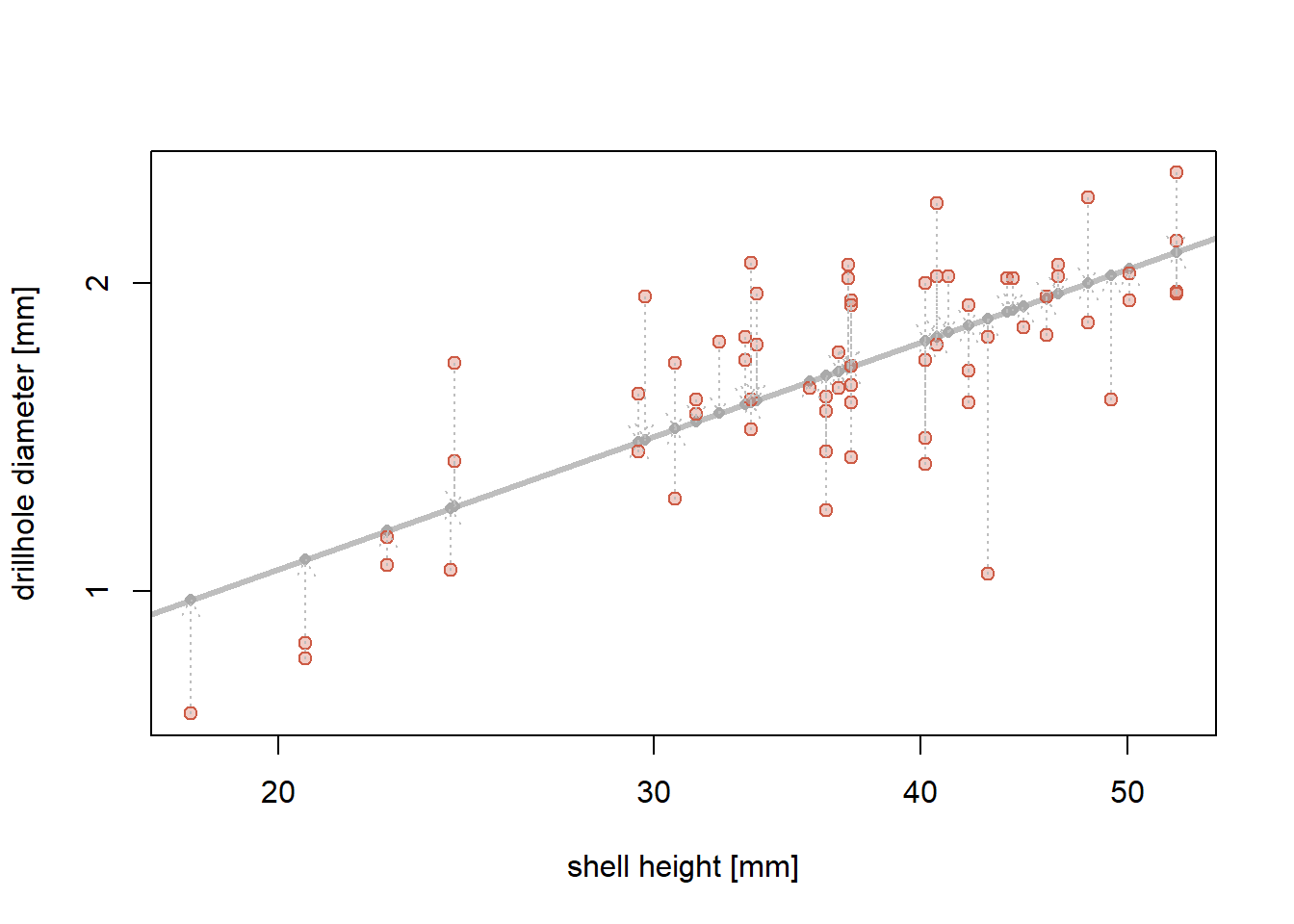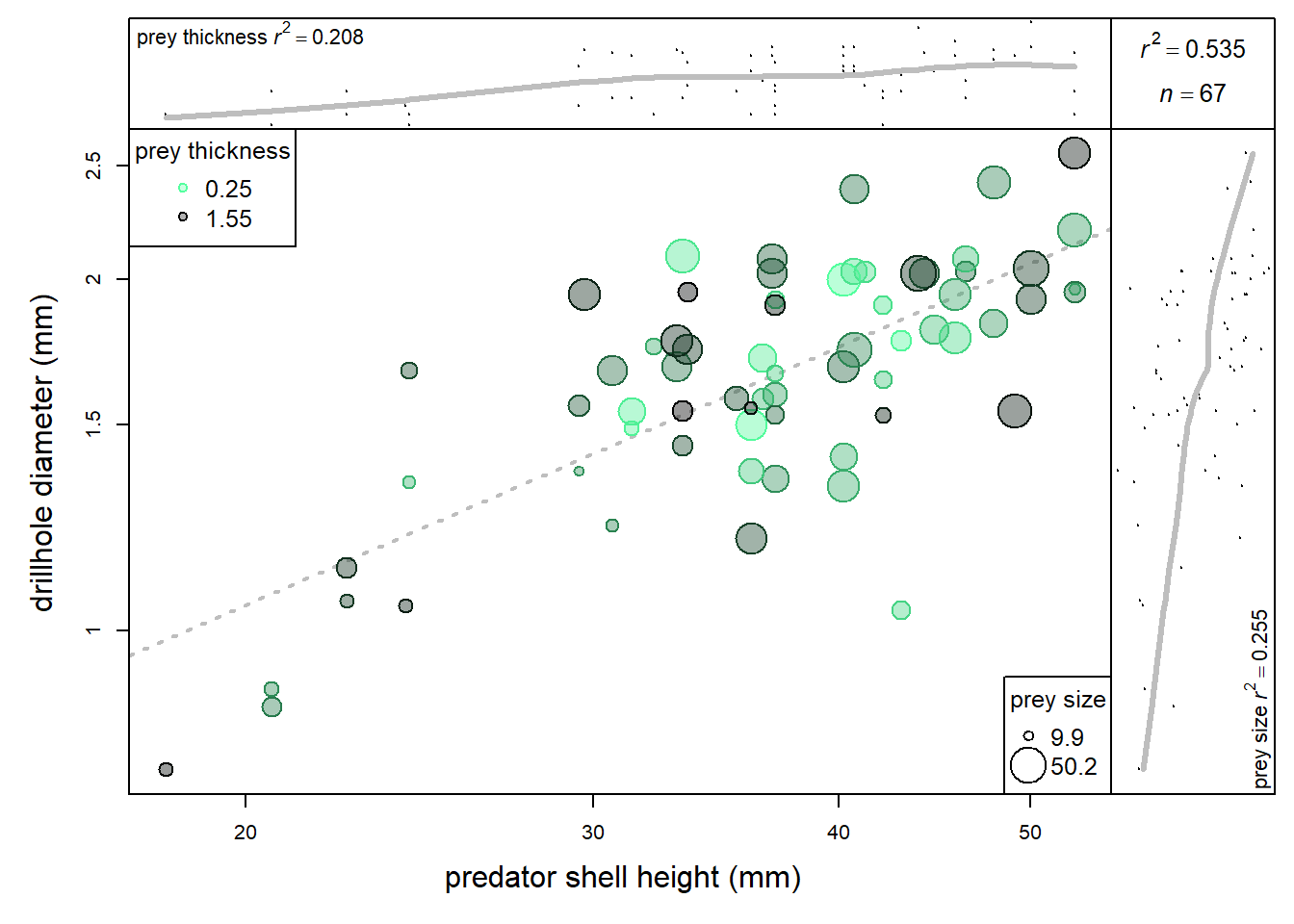We will use data killersnails.csv to explore various types of simple linear regressions. This dataset, based on lab experiments involving predatory snails and mussel prey, includes a series of linear measurements describing shell dimensions of predators, prey, and traces (drillholes) produced by predators when attacking the prey.

options(digits = 3)                             # let's round all outputs to three digits
snails <- read.csv('killersnails.csv', na.strings = '.')    # read the external file (note that missing values are '.' in this case)
snails2 <- na.omit(snails)                      # remove rows with missing values
# install.packages('lmodel2')  # NOT RUN: activate this line to install lmodel2 package

# store variables as follows:
X <- snails2$PredHeight # variable estimating predator size Y <- snails2$DrillMaxOuter  # variable estimating drill hole size)
Z <- snails2$PreyWidth # variable estimating prey size V <- snails2$PreyThickAnter # variable estimating prey shell thickness

Step 1. Log-transform all variables using natural log

X <- log(X); Y <- log(Y); Z <- log(Z); V <- log(V)

Step 2. Compute and plot OLS (Ordinary Least-Square Regression), MA (Major Axis Regression), and SMA (Standardized Major Axis Regression - also knows as Reduced Major Axis Regression) for ‘DrillMaxOuter’ (dependent Y) and ‘PredHeight’ (independent X) using custom-written statements and assemble those results into a 3 by 2 table.

#OLS
slopeOLS <- cov(X, Y) / var(X)              # compute slope coefficient
interceptOLS <- mean(Y) - slopeOLS * mean(X)        # compute intercept

#MA
slopeMA <- 0.5 * (var(Y) / cov(X,Y) - var(X) / cov(X,Y)+
sign(cov(X, Y)) * (4 + (var(Y) / cov(X,Y) - var(X) / cov(X,Y))^2)^0.5)
interceptMA <- mean(Y) - slopeMA * mean(X)

#SMA
slopeSMA <- sign(cov(X, Y)) * sd(Y) / sd(X)
interceptSMA <- mean(Y) - slopeSMA * mean(X)
( my.reg <- rbind(OLS = c(intercept = interceptOLS, slope = slopeOLS),
MA = c(interceptMA, slopeMA), SMA = c(interceptSMA, slopeSMA)) )
##     intercept slope
## OLS     -2.15 0.734
## MA      -3.12 1.005
## SMA     -3.12 1.004

Step 3. Compute slope and intercept values again using lmodel2 package and then assemble those results into a 3 by 2 table (extract only the six values you need here). Note that the results are identical to those obtained in step 2.

( lm2.reg <- lmodel2(Y ~ X)$regression.results[,1:3] ) ## RMA was not requested: it will not be computed. ## No permutation test will be performed ## Method Intercept Slope ## 1 OLS -2.15 0.734 ## 2 MA -3.12 1.005 ## 3 SMA -3.12 1.004 Step 4. Compute predicted values and OLS residuals for Y (drillhole diameter). A few top rows of the resulting output ‘resY’ are printed below. predY <- slopeOLS*X + interceptOLS resY <- Y - predY head(cbind(predicted = predY, residuals = resY)) # check out a few top rows of 'resY' file ## predicted residuals ## [1,] 0.504 0.1483 ## [2,] 0.504 -0.0403 ## [3,] 0.472 -0.0142 ## [4,] 0.494 0.0425 ## [5,] 0.494 -0.0366 ## [6,] 0.400 0.1596 Step 5. Plot X-Y scatter plot, including regression line. Also plot predicted Y values on the regression line (gray points). Visualize magnitude of residuals with vertical arrows connecting each observation to its predicted value. Replace log values on plot axes with non-log values (to make it easier on readers). Use semi-transparent symbols. plot(X, Y, type = 'n', las = 1, xlab = 'shell height [mm]', ylab = 'drillhole diameter [mm]', axes = F) abline(a = interceptOLS, b = slopeOLS, lwd = 3, col = 'gray') points(X, predY, pch = 16, cex = 0.8, col = 'darkgray') points(X, Y, pch = 21, col = 'coral3', bg = adjustcolor('coral3', 0.3)) arrows(X, Y, X, predY, lwd = 1, lty = 3, col = 'gray', length = 0.1) axis(2, at=c(log(1), log(2)), labels=c(1, 2)) axis(1, at=c(log(20), log(30), log(40), log(50)), labels=c(20, 30, 40, 50)) box()Step 6. Compute Perason correlation of residuals from the OLS regression against the variables V and Z (assess statistical significance of r against H: r = 0). Assemble into a 2 x 3 table reporting r, t, and p for both Z and V. corZ <- cor.test(resY, Z) corV <- cor.test(resY, V) rbind(Z=c(corZ$estimate, corZ$statistic, p=corZ$p.value),
V=c(corV$estimate, corV$statistic, p=corV$p.value)) ## cor t p ## Z 0.217 1.79 0.0777 ## V 0.169 1.38 0.1713 Step 7. Make a plot of predator size (X) versus drillhole diameter (X) and incorporate visually other two variables (V and Z). # define a color gradient mycol.F <- colorRampPalette(c("seagreen1", "black")) # this function creates a function mycols <- mycol.F(length(X)) # usw function to create color codes along a color gradient mycolsV <- mycols[order(V)] # order color codes by variable V # define a multi-panel plot using layout par(mai = c(0, 0, 0, 0), omi = c(0.7, 0.7, 0.1, 0.1), xpd = T) p.a <- c(rep(1, 12), rep(2, 2), rep(1, 12), rep(2, 2), rep(c(rep(3, 12), c(4, 4)), 12)) layout(matrix(p.a, 14, 14, byrow = T)) plot(V ~ X, main = '', axes = F, cex = 0.2) VX <- loess(V ~ X) j <- order(X) lines(X[j], VX$fitted[j], col="gray", lwd=3)
mtext(side = 3, line = -1.4, cex=0.7, adj=0.01,
bquote('prey thickness'~italic(r)^2 == .(cor(Y,V)^2)))
box()

plot(0, 0, axes = F, type = 'n')
mtext(side = 3, line = -1.8, bquote(italic(r)^2 == .(round(cor(X,Y)^2,3))), cex=0.8)
mtext(side = 3, line = -3.6, bquote(italic(n) == .(length(X))), cex=0.8)
box()

plot(X, Y, type='n', las=1, xlab='', ylab='drillhole diameter', axes=F)
abline(a=interceptOLS, b=slopeOLS, lwd=2, lty=3, col='gray')
points(X, Y, pch=21, col=mycolsV, cex=1.8*Z-3, bg=adjustcolor(mycolsV, 0.4))
axis(2, at=c(log(1), log(1.5), log(2), log(2.5)), labels=c(1, 1.5, 2, 2.5))
axis(1, at=c(log(20), log(30), log(40), log(50)), labels=c(20, 30, 40, 50))
legend('bottomright', pch=21, pt.cex=range(1.8*Z-3), as.character(exp(range(Z))), cex=1.2, title='prey size')
as.character(exp(range(V))), cex=1.2, title='prey thickness')
box()

plot(Y ~ Z, cex=0.2, main='', axes=F)
YZ <- loess(Z ~ Y)
j2 <- order(Y)
lines(YZ\$fitted[j2], Y[j2], col="gray",lwd=3)
mtext(side=4, line=-1, bquote('prey size'~italic(r)^2 == .(cor(Y,Z)^2)), cex=0.7, adj=0.01)
box()
mtext(side=1, line=3, adj=0.4, outer=T, 'predator shell height (mm)')
mtext(side=2, line=3, adj=0.4, outer=T, 'drillhole diameter (mm)')Citations (always acknowledge packages you use in your scripts)

citation('lmodel2')
##
## To cite package 'lmodel2' in publications use:
##
##   Pierre Legendre (2018). lmodel2: Model II Regression. R package
##   version 1.7-3. https://CRAN.R-project.org/package=lmodel2
##
## A BibTeX entry for LaTeX users is
##
##   @Manual{,
##     title = {lmodel2: Model II Regression},
##     author = {Pierre Legendre},
##     year = {2018},
##     note = {R package version 1.7-3},
##     url = {https://CRAN.R-project.org/package=lmodel2},
##   }
##
## ATTENTION: This citation information has been auto-generated from
## the package DESCRIPTION file and may need manual editing, see
## 'help("citation")'.